## Excel RAND Function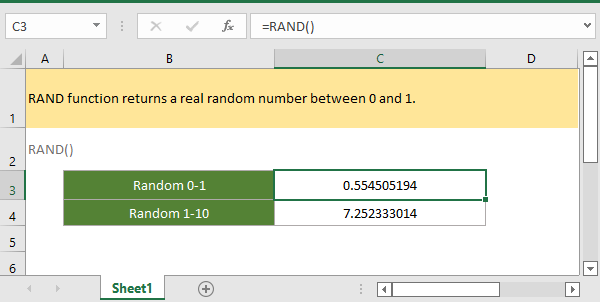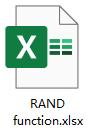### Description

The Excel RAND function returns a random real number between 0 and 1. The result excludes 0 and 1. Every time RAND function returns a new random number when the worksheet is changed or opened.

### Syntax and arguments

Formula syntax

RAND()

Arguments

RAND function has no arguments.

Return Value

The RAND function returns to a real number.

Remarks

1. The RAND function calculates a new result when the worksheet is changed, if you want to stop the result changing, copy and paste the result as a value only to convert it to a static result.

2. If you want to use the random number which is returned by RAND function into other formulas, for stopping the result changed by pressing Enter key, you can use F9 key to get the result, it will change the formula result to a static value.

3. RAND function returns a real number less than 1 and greater than 0.

Version

Excel 2003

### Usage and Examples

Example 1: basic usage

To get a random number between 0 and 1, please select a cell and type the formula:

=RAND()

Press Enter key to get the random number.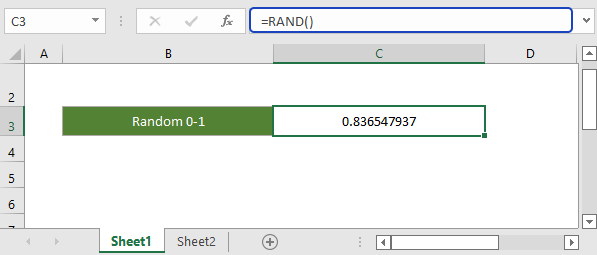Example 2: get random number between two values

The RAND function also can get a random real number between two specified values.

General formula

=RAND()*(b-a)+a

b is the top one in the value range, a is the bottom one, the returned result less than b, but more than a.

To get a random number between 0 and 1, please select a cell and type the formula:

=RAND()*(10-1)+1

Press Enter key to get the random number.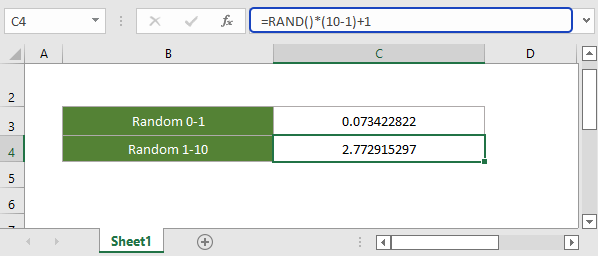Example 3: get random numbers in a selected range

If you want to get random numbers in a selected range at once time, please select a range, and type the RAND function in Formula Bar, then hold Ctrl and press Enter key.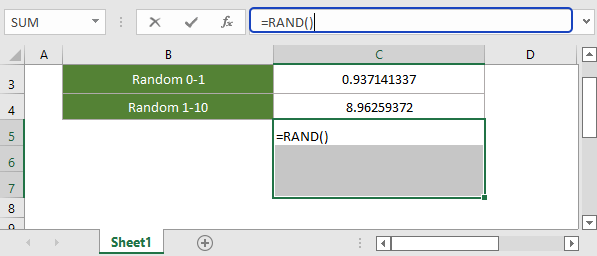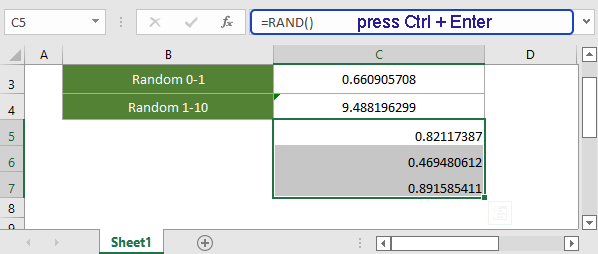#### Relative Functions:

• Excel FACT Function
The FACT function eturns the factorial of a given number. For example, FACT(5)=120, equivalent to 5 x 4 x 3 x 2 x 1.

• Excel FACTDOUBLE Function
The FACTDOUBLE function returns the double factorial of a given number. For example, FACTDOUBLE(5)=15, equivalent 5 x 3 x 1.

• Excel FLOOR Function
The FLOOR function rounds a given number down to the nearest multiple of the specified significance.

• Excel FLOOR.MATH Function
The Excel FLOOR.MATH function rounds a given number down to the nearest integer or nearest multiple of the specified significance.

### The Best Office Productivity Tools

#### Kutools for Excel - Helps You To Stand Out From Crowd

Would you like to complete your daily work quickly and perfectly? Kutools for Excel brings 300 powerful advanced features (Combine workbooks, sum by color, split cell contents, convert date, and so on...) and save 80% time for you.

• Designed for 1500 work scenarios, helps you solve 80% Excel problems.
• Reduce thousands of keyboard and mouse clicks every day, relieve your tired eyes and hands.
• Become an Excel expert in 3 minutes. No longer need to remember any painful formulas and VBA codes.
• 30-day unlimited free trial. 60-day money back guarantee. Free upgrade and support for 2 years.#### Office Tab - Enable Tabbed Reading and Editing in Microsoft Office (include Excel)

• One second to switch between dozens of open documents!
• Reduce hundreds of mouse clicks for you every day, say goodbye to mouse hand.
• Increases your productivity by 50% when viewing and editing multiple documents.
• Brings Efficient Tabs to Office (include Excel), Just Like Chrome, Firefox, And New Internet Explorer.